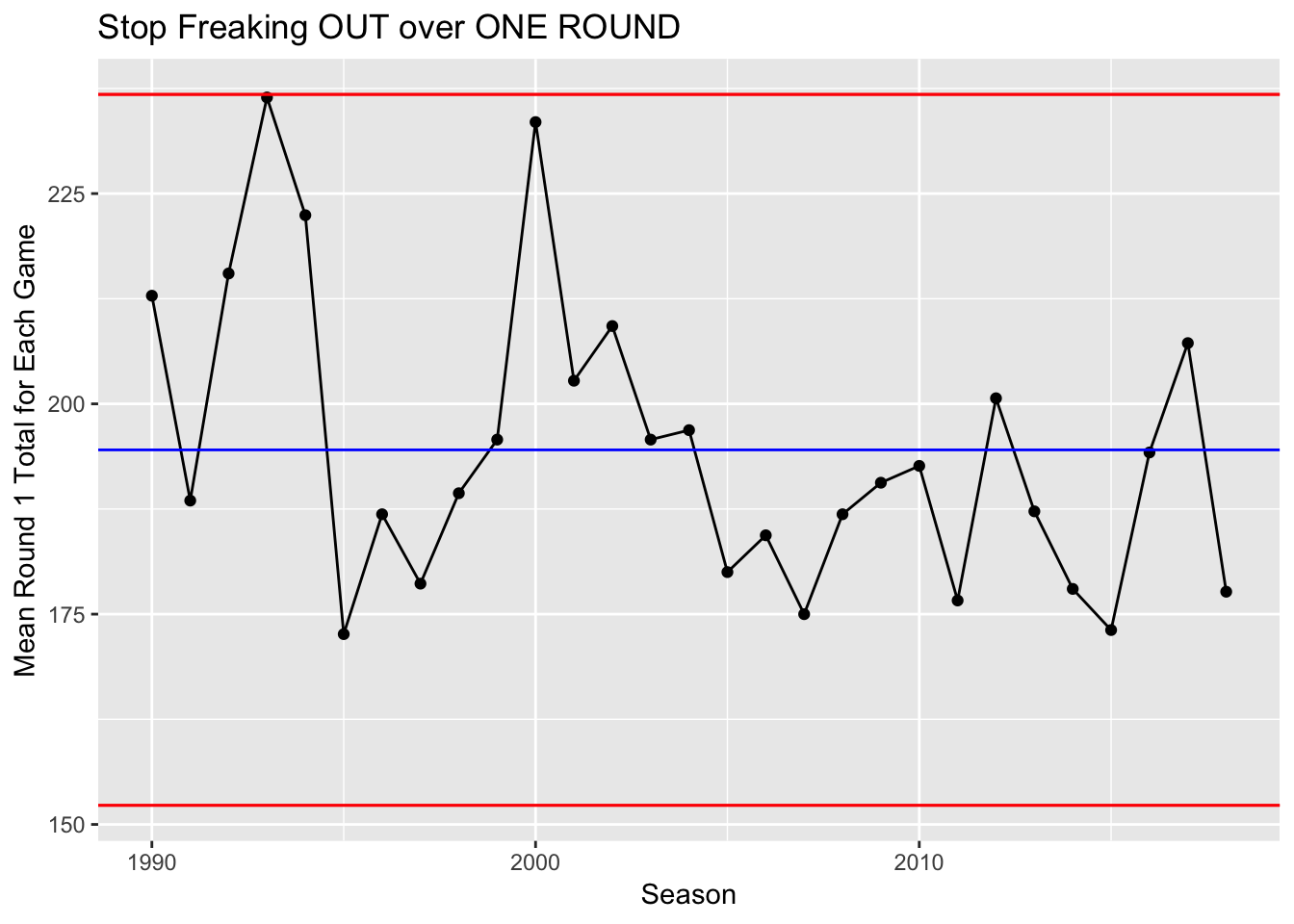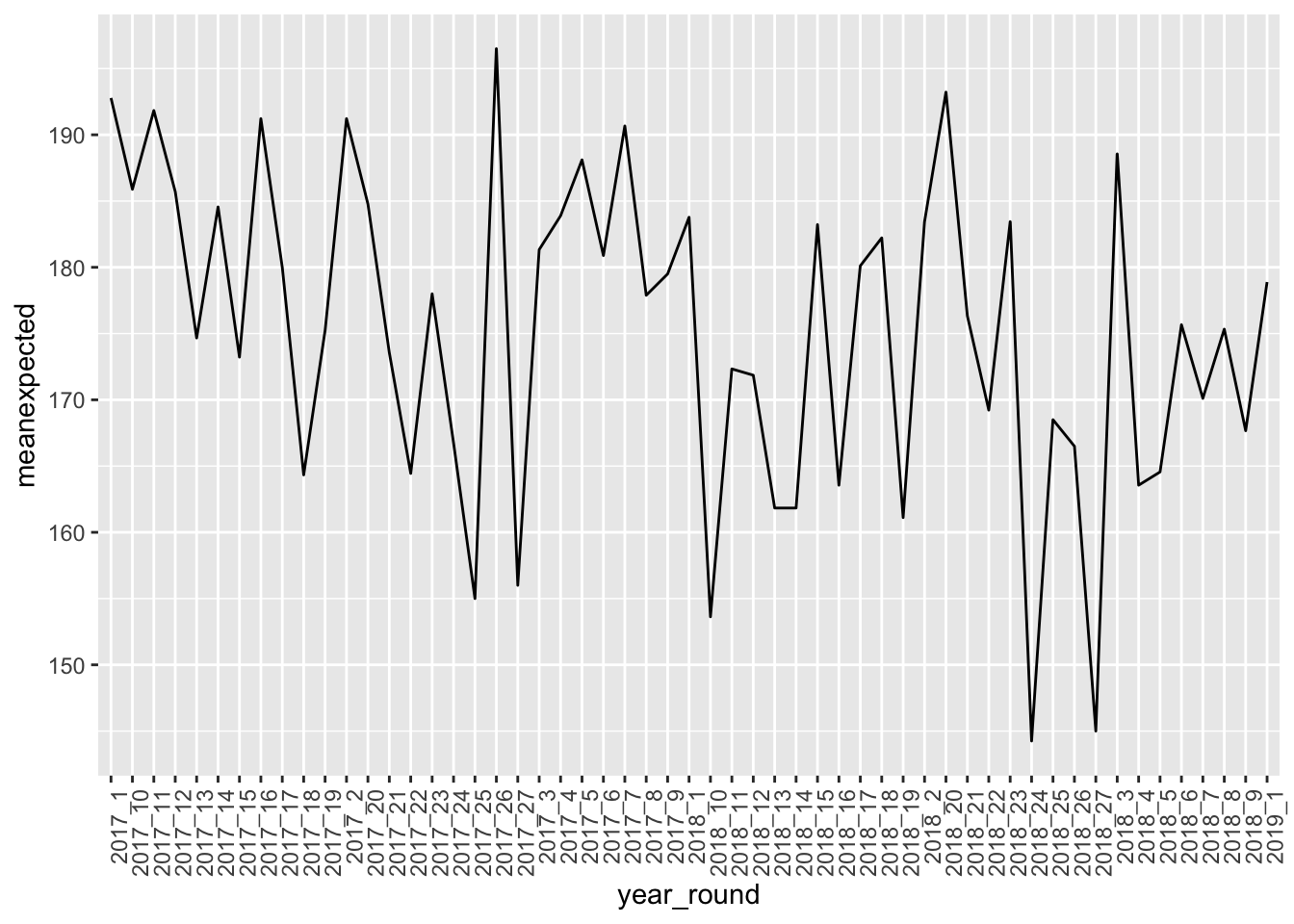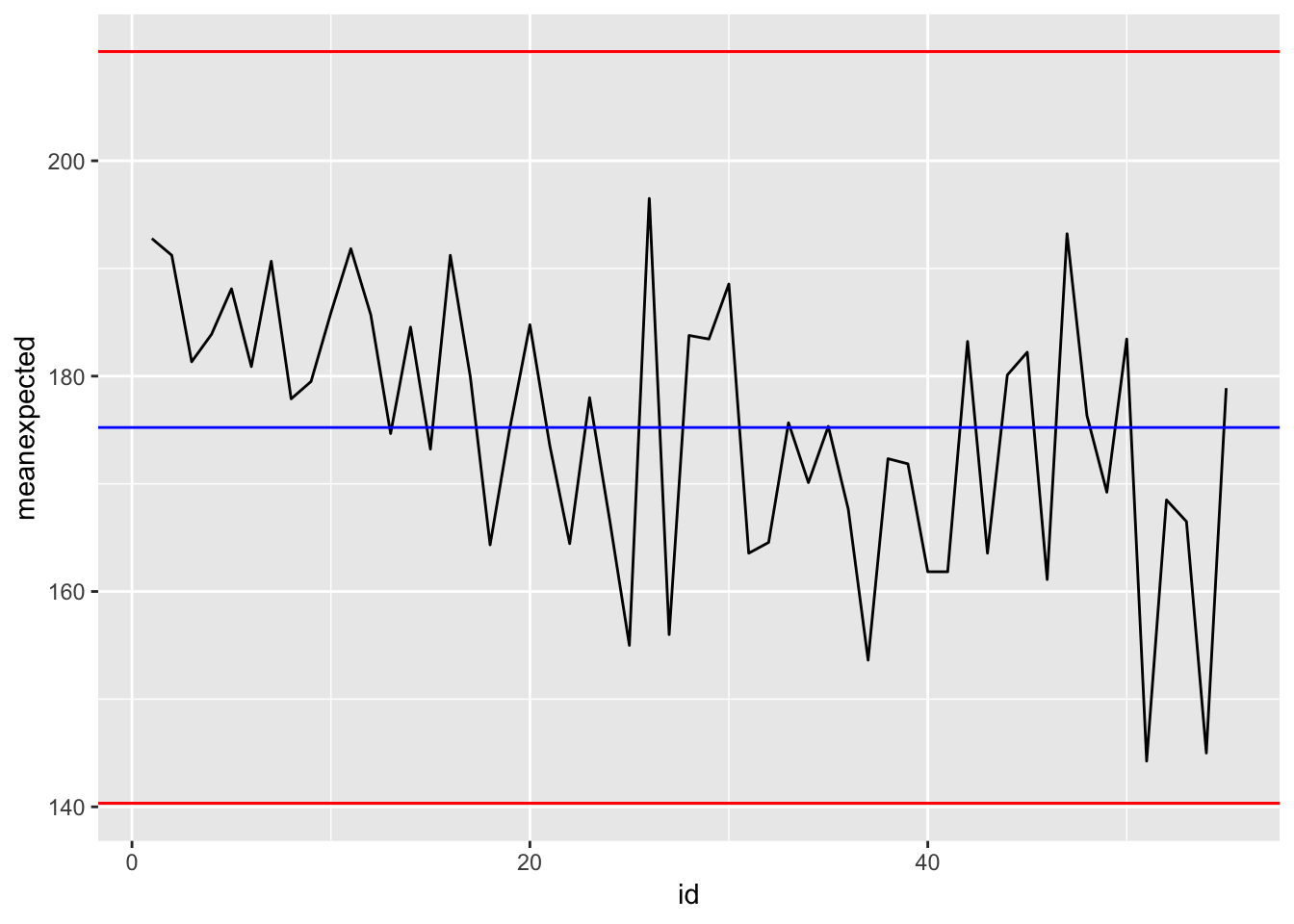Who doesn’t like a wikipedia entry control chart If analysis of the control chart indicates that the process is currently under control (i.e., is stable, with variation only coming from sources common to the process), then no corrections or changes to process control parameters are needed or desired I mean gee whiz this sure could relate to something like I don’t know AFL total game scores?

There seems to always be talk about the scores in AFLM see AFL website, foxsports just to name a couple. Of course you could find more if you searched out for it as well.

Let’s use fitzRoy and the good people over at statsinsder who have kindly provided me with the expected score data you can get from the herald sun.

First thing, lets use fitzRoy

library(fitzRoy)
library(tidyverse)
## ── Attaching packages ──────────────── tidyverse 1.2.1 ──
## ✔ ggplot2 3.1.0       ✔ purrr   0.3.2
## ✔ tibble  2.1.1       ✔ dplyr   0.8.0.1
## ✔ tidyr   0.8.3       ✔ stringr 1.4.0
## ✔ readr   1.3.1       ✔ forcats 0.4.0
## ── Conflicts ─────────────────── tidyverse_conflicts() ──
## ✖ dplyr::lag()    masks stats::lag()
library(ggQC)
fitzRoy::match_results%>%
mutate(total=Home.Points+Away.Points)%>%
group_by(Season,Round)%>%
summarise(meantotal=mean(total))%>%
filter(Season>1989 &  Round=="R1")%>%
ggplot(aes(x=Season,y=meantotal))+geom_point()+geom_line()+stat_QC(method="XmR")+ylab("Mean Round 1 Total for Each Game") +ggtitle("Stop Freaking OUT over ONE ROUND")So if we were to look at the control chart just for round 1 in each AFLM season since the 90s it would seem as though that even though this round was lower scoring that there isn’t much too see here.

After all we can and should expect natural variation in scores, wouldn’t footy be boring if scores were the same every week.

So next lets thing about the expected scores framework

So the thing is we know that week to week thanks to matterofstats we know that scoring does have certain properties. But what I want to look at with a control chart is last week in round 1 2019, were team creating worse oppotunities and outside the bounds that would cause us to worry if we were using a control chart at work.

## # A tibble: 6 x 8
##   Home_Team   Away_Team      Year Round H_CD_Exp A_CD_Exp H_Actual A_Actual
##   <chr>       <chr>         <dbl> <dbl>    <dbl>    <dbl>    <dbl>    <dbl>
## 1 Carlton     Richmond       2017     1       70      120       89      132
## 2 Collingwood Western Bull…  2017     1       91       88       86      100
## 3 Sydney      Port Adelaide  2017     1       79       90       82      110
## 4 St Kilda    Melbourne      2017     1       99      109       90      120
## 5 Gold Coast  Brisbane Lio…  2017     1       73       98       96       98
## 6 Essendon    Hawthorn       2017     1      117       90      116       91

So now lets get plotting.

CDExp_17%>%
mutate(totalexpected=H_CD_Exp + A_CD_Exp)%>%
group_by(Year, Round)%>%
summarise(meanexpected=mean(totalexpected))%>%
unite("year_round", Year, Round)%>%
ggplot(aes(x=year_round, y=meanexpected, group=1))+
geom_line()+
theme(axis.text.x = element_text(angle = 90, hjust = 1))So what we can see here is that the plot has been re-ordered even though our dataframe is in the order that we want.

CDExp_17%>%
mutate(totalexpected=H_CD_Exp + A_CD_Exp)%>%
group_by(Year, Round)%>%
summarise(meanexpected=mean(totalexpected))%>%
unite("year_round", Year, Round)
## # A tibble: 55 x 2
##    year_round meanexpected
##    <chr>             <dbl>
##  1 2017_1             193.
##  2 2017_2             191.
##  3 2017_3             181.
##  4 2017_4             184.
##  5 2017_5             188.
##  6 2017_6             181.
##  7 2017_7             191.
##  8 2017_8             178.
##  9 2017_9             180.
## 10 2017_10            186.
## # … with 45 more rows

So what is going on here is that ggplot orders the graph by the levels of our dataframe

df<-CDExp_17%>%
mutate(totalexpected=H_CD_Exp + A_CD_Exp)%>%
group_by(Year, Round)%>%
summarise(meanexpected=mean(totalexpected))%>%
unite("year_round", Year, Round)
str(df)
## Classes 'tbl_df', 'tbl' and 'data.frame':    55 obs. of  2 variables:
##  $year_round : chr "2017_1" "2017_2" "2017_3" "2017_4" ... ##$ meanexpected: num  193 191 181 184 188 ...
df$year_round<-as.factor(df$year_round)
levels(df\$year_round)
##   "2017_1"  "2017_10" "2017_11" "2017_12" "2017_13" "2017_14" "2017_15"
##   "2017_16" "2017_17" "2017_18" "2017_19" "2017_2"  "2017_20" "2017_21"
##  "2017_22" "2017_23" "2017_24" "2017_25" "2017_26" "2017_27" "2017_3"
##  "2017_4"  "2017_5"  "2017_6"  "2017_7"  "2017_8"  "2017_9"  "2018_1"
##  "2018_10" "2018_11" "2018_12" "2018_13" "2018_14" "2018_15" "2018_16"
##  "2018_17" "2018_18" "2018_19" "2018_2"  "2018_20" "2018_21" "2018_22"
##  "2018_23" "2018_24" "2018_25" "2018_26" "2018_27" "2018_3"  "2018_4"
##  "2018_5"  "2018_6"  "2018_7"  "2018_8"  "2018_9"  "2019_1"

Which if you notice is the oder of our plot earlier.

So how do we fix that?

Lets just add a numeric column that is the row numbers and that will become our x-axis.

CDExp_17%>%
mutate(totalexpected=H_CD_Exp + A_CD_Exp)%>%
group_by(Year, Round)%>%
summarise(meanexpected=mean(totalexpected))%>%
unite("year_round", Year, Round)%>%
mutate(id = row_number())%>%
ggplot(aes(x=id, y=meanexpected, group=1))+
geom_line()+
stat_QC(method="XmR")There you go, if we think about expected scores as oppotunities created, round 1 was actually on the up from the finals series, players just didn’t kick that well. But hey as we know goal kicking isn’t going to happen at 100% of the time.